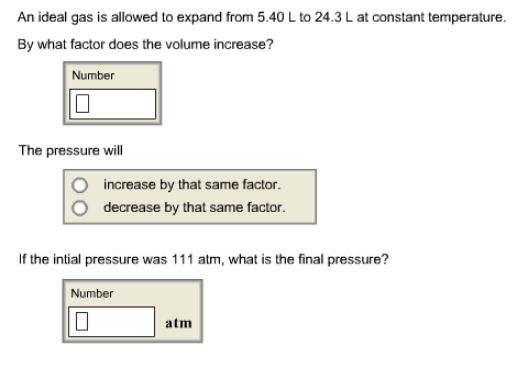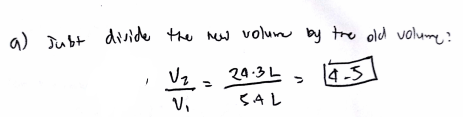Chemistry Practice Problems Chemistry Gas Laws Practice Problems Solution: An ideal gas is allowed to expand from 5.40 L to 2...

🤓 Based on our data, we think this question is relevant for Professor TBD's class at University of Massachusetts Boston.

# Solution: An ideal gas is allowed to expand from 5.40 L to 24.3 Lat constant temperature. By what factor does the volume increase? The pressure will                         increase by that same factor.                         decrease by that same factor If the initial pressure was 111 atm, what is the final pressure?

###### Problem
An ideal gas is allowed to expand from 5.40 L to 24.3 Lat constant temperature.

By what factor does the volume increase?

The pressure will
increase by that same factor.
decrease by that same factor

If the initial pressure was 111 atm, what is the final pressure?###### SolutionView Complete Written Solution

Chemistry Gas Laws

Chemistry Gas Laws

#### Q. A sample of gas with a volume of 3.25 L and a pressure of 785 torr is compressed to a volume of 1.30 L at constant temperature. What is the new pressu...

Solved • Fri Jun 08 2018 14:42:13 GMT-0400 (EDT)

Chemistry Gas Laws

#### Q. A certain mass of nitrogen gas occupies a volume of 5.66 L at a pressure of 8.57 atm. At what pressure will the volume of this sample be 8.04 L? Assum...

Solved • Tue Jun 05 2018 14:26:20 GMT-0400 (EDT)

Chemistry Gas Laws

#### Q. Assuming all gases are at the same temperature and pressure, how many milliliters of nitrogen gas react to give 47.0 mL of ammonia gas? 3H2(g) + N2(g)...

Solved • Wed May 30 2018 14:36:29 GMT-0400 (EDT)

Chemistry Gas Laws

#### Q. An ideal gas in a sealed container has an initial volume of 2.55 L. At constant pressure, it is cooled to 23.00 °C where its final volume is 1.75 L. W...

Solved • Tue May 29 2018 15:12:04 GMT-0400 (EDT)